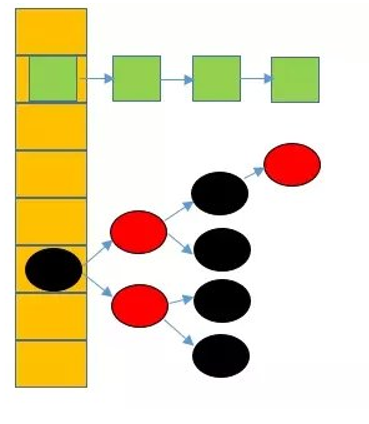# 一、HashMap基础

## 1.1 HashMap的定义

public class HashMap<K,V> extends AbstractMap<K,V> implements Map<K,V>, Cloneable, Serializable

## 1.2 HashMap的属性

//默认容量
static final int DEFAULT_INITIAL_CAPACITY = 1 << 4;
//最大容量
static final int MAXIMUM_CAPACITY = 1 << 30;
//默认加载因子
static final float DEFAULT_LOAD_FACTOR = 0.75f;
//链表转成红黑树的阈值
static final int TREEIFY_THRESHOLD = 8;
//红黑树转为链表的阈值
static final int UNTREEIFY_THRESHOLD = 6;
//存储方式由链表转成红黑树的容量的最小阈值
static final int MIN_TREEIFY_CAPACITY = 64;
//HashMap中存储的键值对的数量
transient int size;
//扩容阈值，当size>=threshold时，就会扩容
int threshold;
//HashMap的加载因子
final float loadFactor;

# 二、初始化

Map<K,V> map = new HashMap<K,V>();

public HashMap(int initialCapacity, float loadFactor) {
if (initialCapacity < 0)
throw new IllegalArgumentException("Illegal initial capacity: " +
initialCapacity);
if (initialCapacity > MAXIMUM_CAPACITY)
initialCapacity = MAXIMUM_CAPACITY;
throw new IllegalArgumentException("Illegal load factor: " +
this.threshold = tableSizeFor(initialCapacity);
}

static final int tableSizeFor(int cap) {
int n = cap - 1;
n |= n >>> 1;
n |= n >>> 2;
n |= n >>> 4;
n |= n >>> 8;
n |= n >>> 16;
return (n < 0) ? 1 : (n >= MAXIMUM_CAPACITY) ? MAXIMUM_CAPACITY : n + 1;
}

cap=19
int n=cap-1;//得到n=18，换算为二进制为10010
n|=n>>>1;//表示n无符号右移一位后，与n按位或计算，其中n>>>1=01001，按位或结果为11011
n|=n>>>2;//其中n>>>2=00110,按位或的结果为11111，下面几步类似，最终得到的结果是n=11111(二进制，也就是2^5-1，31)

# 三、put()

//如果原来存在相同的key-value，原来的value会被替换掉
public V put(K key, V value) {
return putVal(hash(key), key, value, false, true);
}

## 3.1 hash(key)

static final int hash(Object key) {
int h;
return (key == null) ? 0 : (h = key.hashCode()) ^ (h >>> 16);
}

## 3.2 putVal()方法

### 3.2.1 源码

final V putVal(int hash, K key, V value, boolean onlyIfAbsent,
boolean evict) {
Node<K,V>[] tab; Node<K,V> p; int n, i;
if ((tab = table) == null || (n = tab.length) == 0)
n = (tab = resize()).length;
if ((p = tab[i = (n - 1) & hash]) == null)
tab[i] = newNode(hash, key, value, null);
else {
Node<K,V> e; K k;
if (p.hash == hash &&
((k = p.key) == key || (key != null && key.equals(k))))
e = p;
else if (p instanceof TreeNode)
e = ((TreeNode<K,V>)p).putTreeVal(this, tab, hash, key, value);
else {
for (int binCount = 0; ; ++binCount) {
if ((e = p.next) == null) {
p.next = newNode(hash, key, value, null);
if (binCount >= TREEIFY_THRESHOLD - 1) // -1 for 1st
treeifyBin(tab, hash);
break;
}
if (e.hash == hash &&
((k = e.key) == key || (key != null && key.equals(k))))
break;
p = e;
}
}
if (e != null) { // existing mapping for key
V oldValue = e.value;
if (!onlyIfAbsent || oldValue == null)
e.value = value;
afterNodeAccess(e);
return oldValue;
}
}
++modCount;
if (++size > threshold)
resize();
afterNodeInsertion(evict);
return null;
}

• hash：表示key的hash值
• key：待存储的key值
• value：待存储的value值，从这个方法可以知道，HashMap底层存储的是key-value的键值对，不只是存储了value
• onlyIfAbsent：这个参数表示，是否需要替换相同的value值，如果为true，表示不替换已经存在的value
• evict：如果为false，表示数组是新增模式

### 3.2.2 HashMap的数据结构static class Node<K,V> implements Map.Entry<K,V> {
final int hash;
final K key;
V value;
Node<K,V> next;

//省略一些代码

public final int hashCode() {
return Objects.hashCode(key) ^ Objects.hashCode(value);
}

public final V setValue(V newValue) {
V oldValue = value;
value = newValue;
return oldValue;
}

public final boolean equals(Object o) {
if (o == this)
return true;
if (o instanceof Map.Entry) {
Map.Entry<?,?> e = (Map.Entry<?,?>)o;
if (Objects.equals(key, e.getKey()) &&
Objects.equals(value, e.getValue()))
return true;
}
return false;
}
}

### 3.2.3 继续分析putVal()方法

if ((tab = table) == null || (n = tab.length) == 0)
n = (tab = resize()).length;

if ((p = tab[i = (n - 1) & hash]) == null)
tab[i] = newNode(hash, key, value, null);

// Create a regular (non-tree) node
Node<K,V> newNode(int hash, K key, V value, Node<K,V> next) {
return new Node<>(hash, key, value, next);
}

Node<K,V> e; K k;
if (p.hash == hash &&
((k = p.key) == key || (key != null && key.equals(k))))
e = p;

else if (p instanceof TreeNode)
e = ((TreeNode<K,V>)p).putTreeVal(this, tab, hash, key, value);

    for (int binCount = 0; ; ++binCount) {
if ((e = p.next) == null) {
p.next = newNode(hash, key, value, null);
if (binCount >= TREEIFY_THRESHOLD - 1) // -1 for 1st
treeifyBin(tab, hash);
break;
}
if (e.hash == hash &&
((k = e.key) == key || (key != null && key.equals(k))))
break;
p = e;
}

if (e != null) { // existing mapping for key
V oldValue = e.value;
if (!onlyIfAbsent || oldValue == null)
e.value = value;
afterNodeAccess(e);
return oldValue;
}

if (++size > threshold)
resize();